# 协方差深入解读

## 统计学基本概念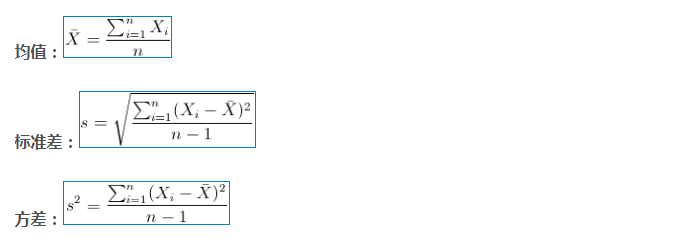## 为什么需要协方差？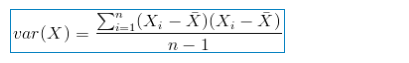## 协方差的意义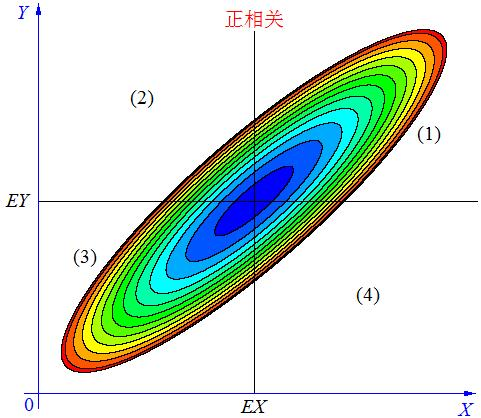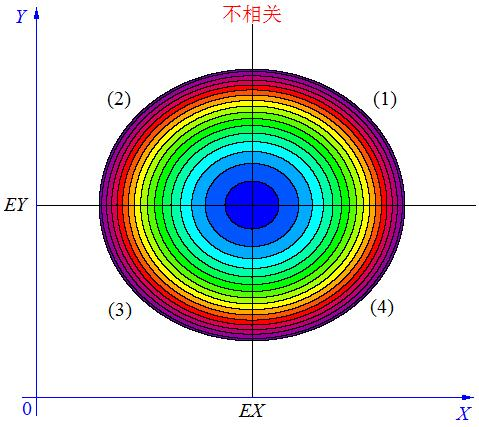cov(X, Y) = E(X-EX)(Y-EY)

## 协方差多了就是协方差矩阵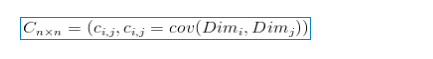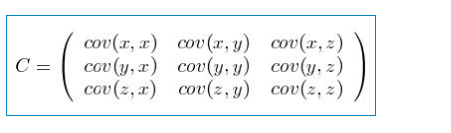## 关于协方差矩阵的解读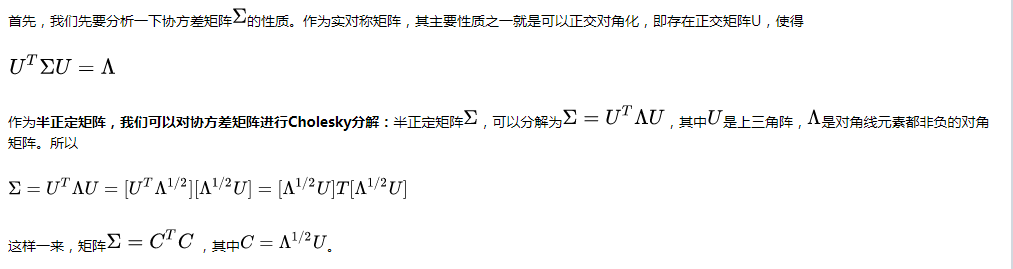### 1.在金融随机分析和金融工程中的应用

Quanto Nikkei Option. Consider a Nikkei quanto into dollar call option. Assuming both the USD/JPY and Nikkei are both lognormal process, i.e.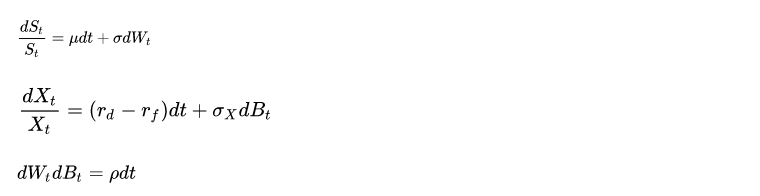where S and X are the equity and FX process. On a spreadsheet, simulate the process, and show that by delta hedging alone, you can replicate the quanto call option. Assume the maturity of the option is one year.

Sigma = [siga^2 siga*sigb*rho;
siga*sigb*rho sigb^2];
B = randn(2,n);
C=chol(Sigma);
V = C' * B;
STa = S0a * exp((mua - (siga^2)/2)*T + sqrt(T)*V(1,:));
STb = S0b * exp((mub - (sigb^2)/2)*T + sqrt(T)*V(2,:));

## Python 中使用

numpy.cov()的作用是计算协方差矩阵，下面给出几个例子

>>> x = np.array([[0, 2], [1, 1], [2, 0]]).T
>>> x
array([[0, 1, 2],
[2, 1, 0]])

>>> np.cov(x)
array([[ 1., -1.],
[-1.,  1.]])